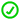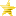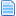Wall bounce error (Read 235 times)

Started by ziaker, May 12, 2019, 12:48:23 am

ziakerWall bounce error
#1  May 12, 2019, 12:48:23 am
•••at 0:05

Code:
`[State 210, 1]type = HitDeftriggerall = !movecontacttrigger1 = 1attr = S, NAanimtype  = Lightdamage    = 120,50guardflag = MApausetime = 12,12sparkno = -1;s6000guard.sparkno = s7000sparkxy = -8,-25hitsound   = S0,13guardsound = S0,8ground.type = Highground.slidetime = 15ground.hittime  = 15ground.velocity = -1,-8air.velocity = -32,-6fall = 1envshake.time = 20envshake.freq = 220envshake.ampl = -10envshake.phase = 120p2stateno = 1790;targetstate = 1790;p2facing = 1`

the character gets stuck in the idle animation

Nep HeartRe: Wall bounce error
#2  May 12, 2019, 12:58:00 am
Think you can post all the wall bounce custom state related code that apparently starts at state 1790 and onward to see what's the problem?

ziakerRe: Wall bounce error
#3  May 12, 2019, 02:13:28 am
•••I found a code in another character and it is now working normal.

Here the code:

;------------------
; Hit by Punt Kick - flying through the air
[Statedef 1591]
type    = A
movetype= H
physics = N

[State 2000, VarAdd - modificación horizontal]
trigger1 = time > 0
v = 10
value = 27

[State 0, AngleDraw]
type = AngleDraw
trigger1 = time > 0
value = var(10)

[State 1291, Velocity]
type = HitVelSet
trigger1 = Time = 0
x = 1
y = 1

[State 1291, Gravity]
trigger1 = 1
y = .45

[State 1291, Hit wall]
type = ChangeState
triggerall = Pos y < -15
trigger1 = BackEdgeBodyDist <= 10
trigger2 = FrontEdgeBodyDist <= 10
value = 1592

[State 1291, Hit ground]
type = SelfState
trigger1 = (Vel y > 0) && (Pos y >= 0)
value = 5100

;------------------
; Hit by Punt Kick - hit wall
[Statedef 1592]
type    = A
movetype= H
physics = N

[State 1292, Turn]
type = Turn
trigger1 = (Time = 0) && (FrontEdgeBodyDist <= 30)

[State 1292, Pos]
trigger1 = Time = 0
x = 15 - BackEdgeBodyDist

[State 1292, Stop moving]
type = PosFreeze
trigger1 = 1
x = 1
y = 1

[State 1292, No scroll]
type = ScreenBound
trigger1 = 1
value = 1
movecamera = 0,1

[State 1292, Spark]
type = Explod
trigger1 = Time = 0
anim = F72
pos = 0, floor (screenpos y) - 50
postype = back
sprpriority = 3

[State 1292, Anim]
type = ChangeAnim2
trigger1 = Time = 0
value = 1027

[State 1292, Sound]
type = PlaySnd
trigger1 = Time = 0
value = F7,0

[State 1292, State]
type = ChangeState
trigger1 = AnimTime = 0
value = 1193

;------------------
; Hit by Punt Kick - bounce off wall
[Statedef 1193]
type    = A
movetype= H
physics = N

[State 1293, Vel Y]
type = VelSet
trigger1 = Time = 0
y = -7

[State 1293, Vel X]
type = VelSet
trigger1 = Time = 0
x = 3

[State 1293, VelMul]
type = VelMul
trigger1 = 1
x = .98

[State 1293, Turn]
type = Turn
trigger1 = (Time = 0) && (BackEdgeDist < 30)

[State 1293, Gravity]
trigger1 = 1
y = .35

[State 1293, Anim 5050]
type = ChangeAnim
trigger1 = Time = 0
trigger1 = !SelfAnimExist(5052)
value = 5050

[State 1293, Anim 5052]
type = ChangeAnim
trigger1 = Time = 0
trigger1 = SelfAnimExist(5052)
value = 5052

[State 1293, Anim 5060]
type = ChangeAnim
trigger1 = Vel Y > -2
trigger1 = Anim = 5050
trigger1 = SelfAnimExist(5060)
persistent = 0
value = 5060

[State 1293, Anim 5062]
type = ChangeAnim
trigger1 = Vel Y > -2
trigger1 = Anim = 5052
trigger1 = SelfAnimExist(5062)
persistent = 0
value = 5062

[State 1293, Hit ground]
type = SelfState
trigger1 = (Vel y > 0) && (Pos y >= 0)
value = 5100

Nep HeartRe: Wall bounce error
#4  May 12, 2019, 02:19:00 am
I am not really keen on manipulating P2's variables in custom state if this is to go by.

[State 2000, VarAdd - modificación horizontal]